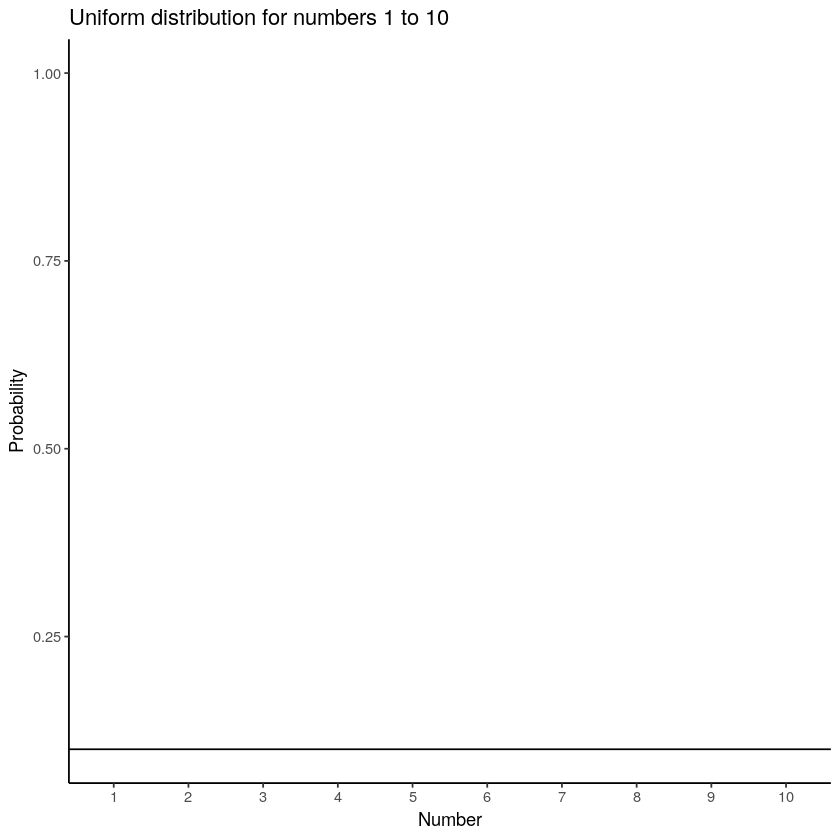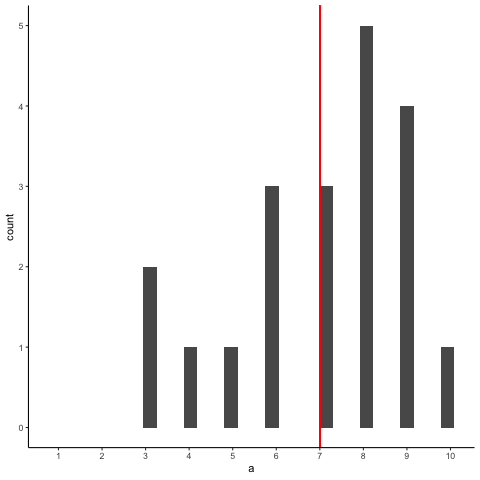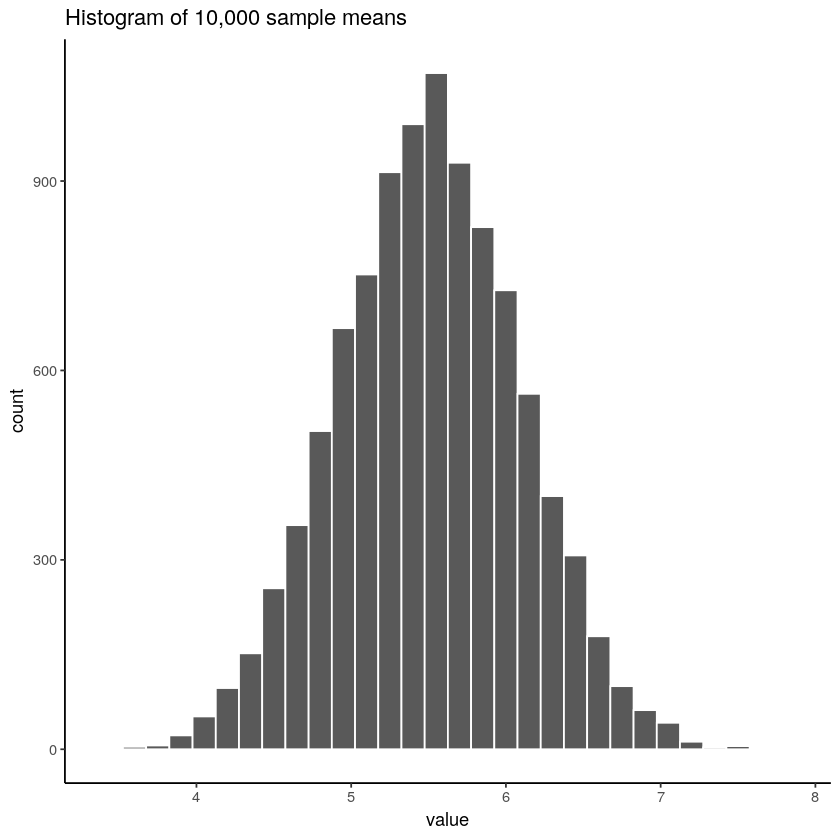# 4.10: Sampling distributions and the central limit theorem

$$\newcommand{\vecs}{\overset { \rightharpoonup} {\mathbf{#1}} }$$ $$\newcommand{\vecd}{\overset{-\!-\!\rightharpoonup}{\vphantom{a}\smash {#1}}}$$$$\newcommand{\id}{\mathrm{id}}$$ $$\newcommand{\Span}{\mathrm{span}}$$ $$\newcommand{\kernel}{\mathrm{null}\,}$$ $$\newcommand{\range}{\mathrm{range}\,}$$ $$\newcommand{\RealPart}{\mathrm{Re}}$$ $$\newcommand{\ImaginaryPart}{\mathrm{Im}}$$ $$\newcommand{\Argument}{\mathrm{Arg}}$$ $$\newcommand{\norm}{\| #1 \|}$$ $$\newcommand{\inner}{\langle #1, #2 \rangle}$$ $$\newcommand{\Span}{\mathrm{span}}$$ $$\newcommand{\id}{\mathrm{id}}$$ $$\newcommand{\Span}{\mathrm{span}}$$ $$\newcommand{\kernel}{\mathrm{null}\,}$$ $$\newcommand{\range}{\mathrm{range}\,}$$ $$\newcommand{\RealPart}{\mathrm{Re}}$$ $$\newcommand{\ImaginaryPart}{\mathrm{Im}}$$ $$\newcommand{\Argument}{\mathrm{Arg}}$$ $$\newcommand{\norm}{\| #1 \|}$$ $$\newcommand{\inner}{\langle #1, #2 \rangle}$$ $$\newcommand{\Span}{\mathrm{span}}$$$$\newcommand{\AA}{\unicode[.8,0]{x212B}}$$

The law of large numbers is a very powerful tool, but it’s not going to be good enough to answer all our questions. Among other things, all it gives us is a “long run guarantee”. In the long run, if we were somehow able to collect an infinite amount of data, then the law of large numbers guarantees that our sample statistics will be correct. But as John Maynard Keynes famously argued in economics, a long run guarantee is of little use in real life:

[The] long run is a misleading guide to current affairs. In the long run we are all dead. Economists set themselves too easy, too useless a task, if in tempestuous seasons they can only tell us, that when the storm is long past, the ocean is flat again. Keynes (1923, 80)

As in economics, so too in psychology and statistics. It is not enough to know that we will eventually arrive at the right answer when calculating the sample mean. Knowing that an infinitely large data set will tell me the exact value of the population mean is cold comfort when my actual data set has a sample size of $$N=100$$. In real life, then, we must know something about the behavior of the sample mean when it is calculated from a more modest data set!

## Sampling distribution of the sample means

“Oh no, what is the sample distribution of the sample means? Is that even allowed in English?”. Yes, unfortunately, this is allowed. The sampling distribution of the sample means is the next most important thing you will need to understand. IT IS SO IMPORTANT THAT IT IS NECESSARY TO USE ALL CAPS. It is only confusing at first because it’s long and uses sampling and sample in the same phrase.

Don’t worry, we’ve been prepping you for this. You know what a distribution is right? It’s where numbers comes from. It makes some numbers occur more or less frequently, or the same as other numbers. You know what a sample is right? It’s the numbers we take from a distribution. So, what could the sampling distribution of the sample means refer to?

First, what do you think the sample means refers to? Well, if you took a sample of numbers, you would have a bunch of numbers…then, you could compute the mean of those numbers. The sample mean is the mean of the numbers in the sample. That is all. So, what is this distribution you speak of? Well, what if you took a bunch of samples, put one here, put one there, put some other ones other places. You have a lot of different samples of numbers. You could compute the mean for each them. Then you would have a bunch of means. What do those means look like? Well, if you put them in a histogram, you could find out. If you did that, you would be looking at (roughly) a distribution, AKA the sampling distribution of the sample means.

“I’m following along sort of, why would I want to do this instead of watching Netflix…”. Because, the sampling distribution of the sample means gives you another window into chance. A very useful one that you can control, just like your remote control, by pressing the right design buttons.

## Seeing the pieces

To make a sampling distribution of the sample means, we just need the following:

1. A distribution to take numbers from
2. A bunch of different samples from the distribution
3. The means of each of the samples
4. Get all of the sample means, and plot them in a histogram

Question

Question for yourself: What do you think the sampling distribution of the sample means will look like? Will it tend to look the shape of the distribution that the samples came from? Or not? Good question, think about it.

Let’s do those four things. We will sample numbers from the uniform distribution, it looks like this if we are sampling from the set of integers from 1 to 10:Figure $$\PageIndex{1}$$: A uniform distribution illustrating the probabilites of sampling the numbers 1 to 10. In a uniform distribution, all numbers have an equal probability of being sampled, so the line is flat indicating all numbers have the same probability.

OK, now let’s take a bunch of samples from that distribution. We will set our sample-size to 20. It’s easier to see how the sample mean behaves in a movie. Each histogram shows a new sample. The red line shows where the mean of the sample is. The samples are all very different from each other, but the red line doesn’t move around very much, it always stays near the middle. However, the red line does move around a little bit, and this variance is what we call the sampling distribution of the sample mean.Figure $$\PageIndex{2}$$: Animiation showing histograms for different samples of size 20 from the uniform distribution. The red line shows the mean of each sample.

OK, what have we got here? We have an animiation of 10 different samples. Each sample has 20 observations and these are summarized in each of histograms that show up in the animiation. Each histogram has a red line. The red line shows you where the mean of each sample is located. So, we have found the sample means for the 10 different samples from a uniform distribution.

First question. Are the sample means all the same? The answer is no. They are all kind of similar to each other though, they are all around five plus or minus a few numbers. This is interesting. Although all of our samples look pretty different from one another, the means of our samples look more similar than different.

Second question. What should we do with the means of our samples? Well, how about we collect them them all, and then plot a histogram of them. This would allow us to see what the distribution of the sample means looks like. The next histogram is just this. Except, rather than taking 10 samples, we will take 10,000 samples. For each of them we will compute the means. So, we will have 10,000 means. This is the histogram of the sample means:Figure $$\PageIndex{3}$$: A histogram showing the sample means for 10,000 samples, each size 20, from the uniform distribution of numbers from 1 to 10. The expected mean is 5.5, and the histogram is centered on 5.5. The mean of each sample is not always 5.5 because of sampling error or chance.

“Wait what? This doesn’t look right. I thought we were taking samples from a uniform distribution. Uniform distributions are flat. THIS DOES NOT LOOK LIKE A FLAT DISTRIBTUION, WHAT IS GOING ON, AAAAAGGGHH.” We feel your pain.

Remember, we are looking at the distribution of sample means. It is indeed true that the distribution of sample means does not look the same as the distribution we took the samples from. Our distribution of sample means goes up and down. In fact, this will almost always be the case for distributions of sample means. This fact is called the central limit theorem, which we talk about later.

For now, let’s talk about about what’s happening. Remember, we have been sampling numbers between the range 1 to 10. We are supposed to get each number with roughly equal frequency, because we are sampling from a uniform distribution. So, let’s say we took a sample of 10 numbers, and happened to get one of each from 1 to 10.

1 2 3 4 5 6 7 8 9 10

What is the mean of those numbers? Well, its 1+2+3+4+5+6+7+8+9+10 = 55 / 10 = 5.5. Imagine if we took a bigger sample, say of 20 numbers, and again we got exactly 2 of each number. What would the mean be? It would be (1+2+3+4+5+6+7+8+9+10)*2 = 110 / 20 = 5.5. Still 5.5. You can see here, that the mean value of our uniform distribution is 5.5. Now that we know this, we might expect that most of our samples will have a mean near this number. We already know that every sample won’t be perfect, and it won’t have exactly an equal amount of every number. So, we will expect the mean of our samples to vary a little bit. The histogram that we made shows the variation. Not surprisingly, the numbers vary around the value 5.5.

## Sampling distributions exist for any sample statistic!

One thing to keep in mind when thinking about sampling distributions is that any sample statistic you might care to calculate has a sampling distribution. For example, suppose that each time you sampled some numbers from an experiment you wrote down the largest number in the experiment. Doing this over and over again would give you a very different sampling distribution, namely the sampling distribution of the maximum. You could calculate the smallest number, or the mode, or the median, of the variance, or the standard deviation, or anything else from your sample. Then, you could repeat many times, and produce the sampling distribution of those statistics. Neat!

Just for fun here are some different sampling distributions for different statistics. We will take a normal distribution with mean = 100, and standard deviation =20. Then, we’ll take lots of samples with n = 50 (50 observations per sample). We’ll save all of the sample statistics, then plot their histograms. Let’s do it:Figure $$\PageIndex{4}$$: Each panel shows a histogram of a different sampling statistic.

We just computed 4 different sampling distributions, for the mean, standard deviation, maximum value, and the median. If you just look quickly at these histograms you might think they all basically look the same. Hold up now. It’s very important to look at the x-axes. They are different. For example, the sample mean goes from about 90 to 110, whereas the standard deviation goes from 15 to 25.

These sampling distributions are super important, and worth thinking about. What should you think about? Well, here’s a clue. These distributions are telling you what to expect from your sample. Critically, they are telling you what you should expect from a sample, when you take one from the specific distribution that we used (normal distribution with mean =100 and SD = 20). What have we learned. We’ve learned a tonne. We’ve learned that we can expect our sample to have a mean somewhere between 90 and 108ish. Notice, the sample means are never more extreme. We’ve learned that our sample will usually have some variance, and that the the standard deviation will be somewhere between 15 and 25 (never much more extreme than that). We can see that sometime we get some big numbers, say between 120 and 180, but not much bigger than that. And, we can see that the median is pretty similar to the mean. If you ever took a sample of 50 numbers, and your descriptive statistics were inside these windows, then perhaps they came from this kind of normal distribution. If your sample statistics are very different, then your sample probably did not come this distribution. By using simulation, we can find out what samples look like when they come from distributions, and we can use this information to make inferences about whether our sample came from particular distributions.

This page titled 4.10: Sampling distributions and the central limit theorem is shared under a CC BY-SA 4.0 license and was authored, remixed, and/or curated by Matthew J. C. Crump via source content that was edited to the style and standards of the LibreTexts platform; a detailed edit history is available upon request.